# Rat in a Maze

Rat in a Maze

Consider a rat placed at (0, 0) in a square matrix of order N * N. It has to reach the destination at (N – 1, N – 1). Find all possible paths that the rat can take to reach from source to destination. The directions in which the rat can move are ‘U'(up), ‘D'(down), ‘L’ (left), ‘R’ (right). Value 0 at a cell in the matrix represents that it is blocked and the rat cannot move to it while value 1 at a cell in the matrix represents that rat can travel through it.

Note: In a path, no cell can be visited more than one time.

Print the answer in lexicographical(sorted) order

Examples:

```Example 1:

Input:
N = 4
m[][] = {{1, 0, 0, 0},
{1, 1, 0, 1},
{1, 1, 0, 0},
{0, 1, 1, 1}}

Output: DDRDRR DRDDRR

Explanation: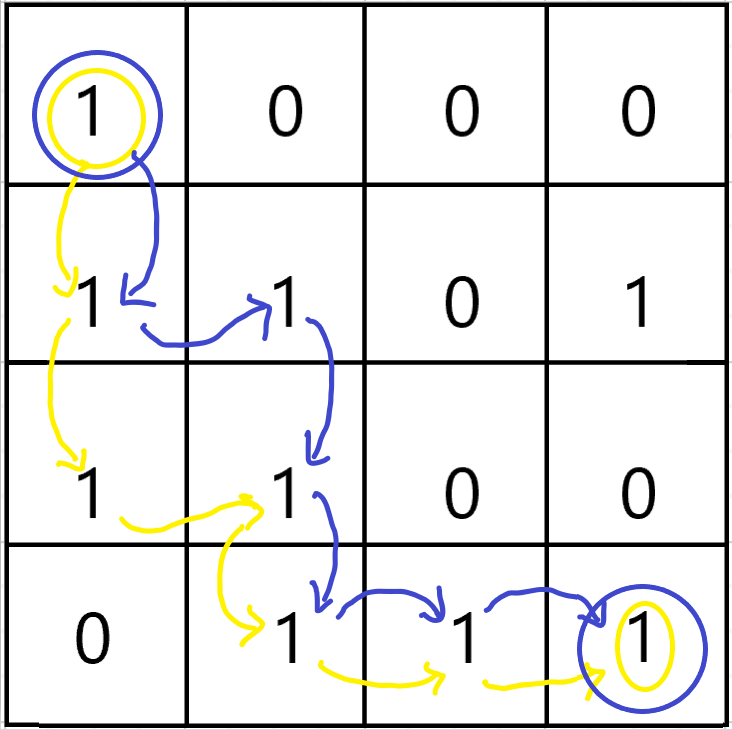The rat can reach the destination at (3, 3) from (0, 0) by two paths - DRDDRR and DDRDRR, when printed in sorted order we get DDRDRR DRDDRR.

Example 2:

Input: N = 2
m[][] = {{1, 0},
{1, 0}}

Output:
No path exists and the destination cell is blocked.
```

### Solution

Disclaimer: Don’t jump directly to the solution, try it out yourself first.

Solution 1: Recursion

Intuition:

The best way to solve such problems is using recursion.

Approach:

• Start at the source(0,0) with an empty string and try every possible path i.e upwards(U), downwards(D), leftwards(L) and rightwards(R).
• As the answer should be in lexicographical order so it’s better to try the directions in lexicographical order i.e (D,L,R,U)
• Declare a 2D-array named visited because the question states that a single cell should be included only once in the path,so it’s important to keep track of the visited cells in a particular path.
• If a cell is in path, mark it in the visited array.
• Also keep a check of the “out of bound” conditions while going in a particular direction in the matrix.
• Whenever you reach the destination(n,n) it’s very important to get back as shown in the recursion tree.
• While getting back, keep on unmarking the visited array for the respective direction.Also check whether there is a different path possible while getting back and if yes, then mark that cell in the visited array.

Recursive tree: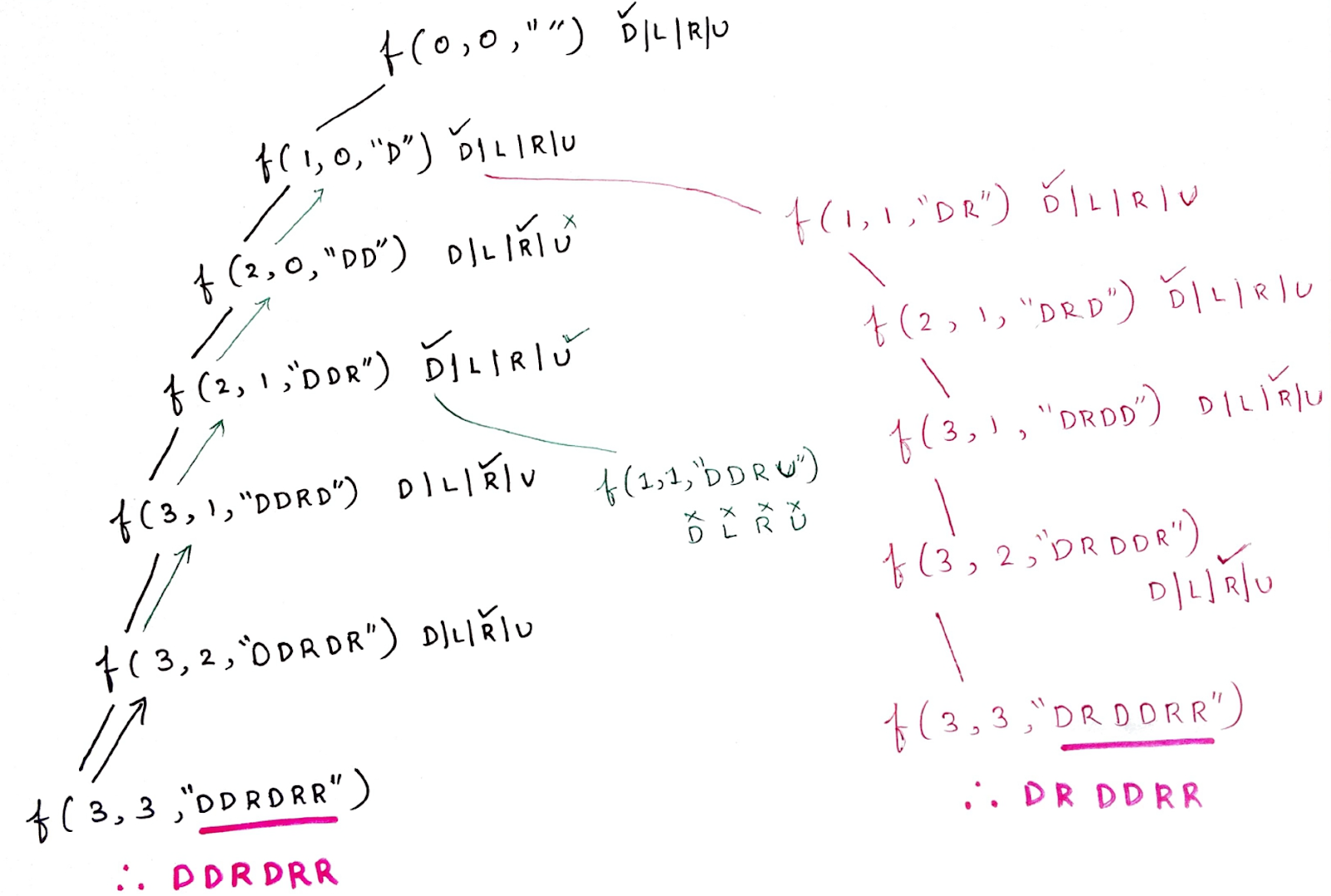For  “DDRDRR” :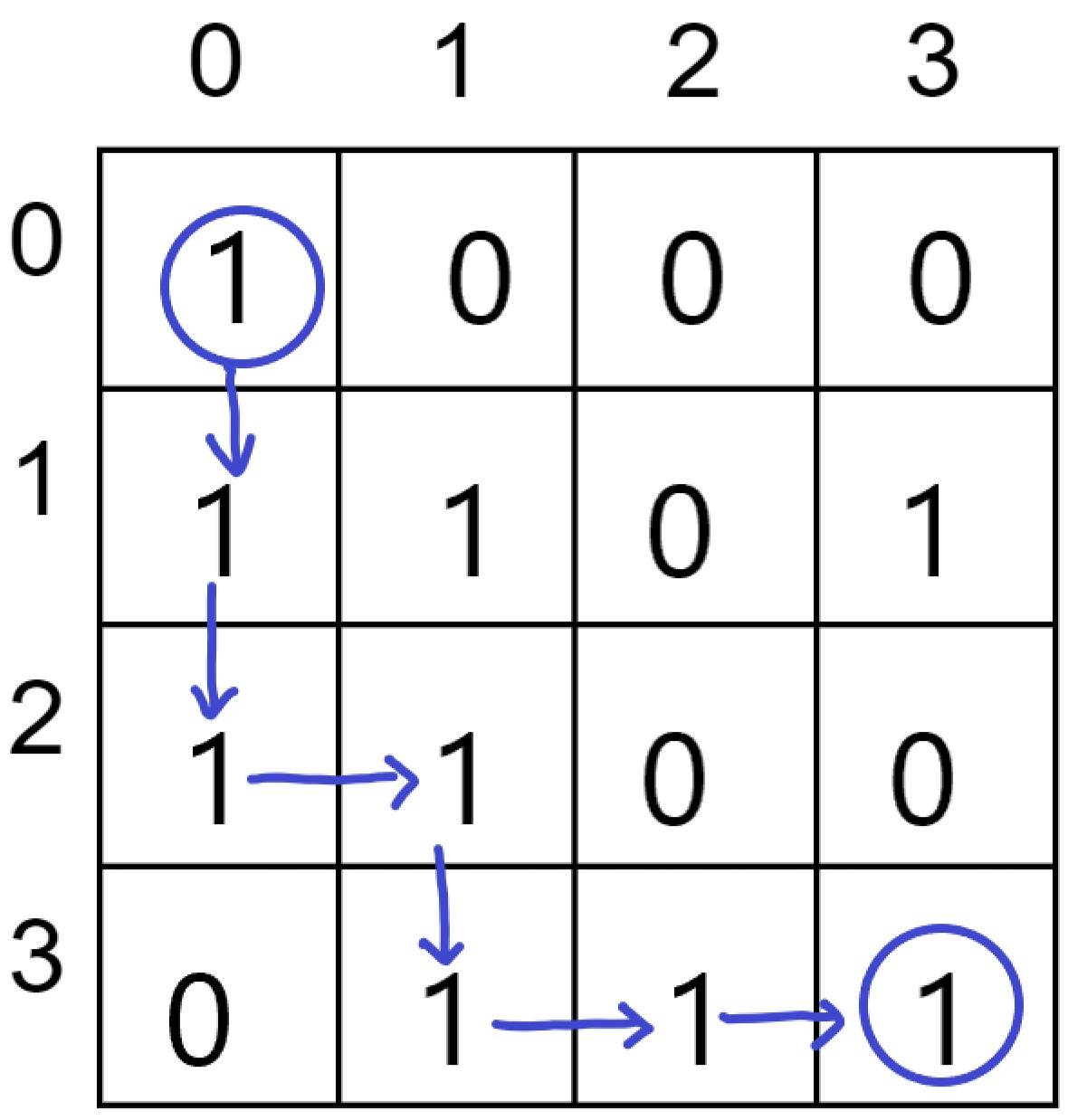Code:

## C++ Code

``````#include <bits/stdc++.h>

using namespace std;

class Solution {
void findPathHelper(int i, int j, vector < vector < int >> & a, int n, vector < string > & ans, string move,
vector < vector < int >> & vis) {
if (i == n - 1 && j == n - 1) {
ans.push_back(move);
return;
}

// downward
if (i + 1 < n && !vis[i + 1][j] && a[i + 1][j] == 1) {
vis[i][j] = 1;
findPathHelper(i + 1, j, a, n, ans, move + 'D', vis);
vis[i][j] = 0;
}

// left
if (j - 1 >= 0 && !vis[i][j - 1] && a[i][j - 1] == 1) {
vis[i][j] = 1;
findPathHelper(i, j - 1, a, n, ans, move + 'L', vis);
vis[i][j] = 0;
}

// right
if (j + 1 < n && !vis[i][j + 1] && a[i][j + 1] == 1) {
vis[i][j] = 1;
findPathHelper(i, j + 1, a, n, ans, move + 'R', vis);
vis[i][j] = 0;
}

// upward
if (i - 1 >= 0 && !vis[i - 1][j] && a[i - 1][j] == 1) {
vis[i][j] = 1;
findPathHelper(i - 1, j, a, n, ans, move + 'U', vis);
vis[i][j] = 0;
}

}
public:
vector < string > findPath(vector < vector < int >> & m, int n) {
vector < string > ans;
vector < vector < int >> vis(n, vector < int > (n, 0));

if (m == 1) findPathHelper(0, 0, m, n, ans, "", vis);
return ans;
}
};

int main() {
int n = 4;

vector < vector < int >> m = {{1,0,0,0},{1,1,0,1},{1,1,0,0},{0,1,1,1}};

Solution obj;
vector < string > result = obj.findPath(m, n);
if (result.size() == 0)
cout << -1;
else
for (int i = 0; i < result.size(); i++) cout << result[i] << " ";
cout << endl;

return 0;
}``````

Output:

DDRDRR DRDDRR

Time Complexity: O(4^(m*n)), because on every cell we need to try 4 different directions.

Space Complexity:  O(m*n) ,Maximum Depth of the recursion tree(auxiliary space).

## Java Code

``````import java.util.*;

// m is the given matrix and n is the order of matrix
class Solution {
private static void solve(int i, int j, int a[][], int n, ArrayList < String > ans, String move,
int vis[][]) {
if (i == n - 1 && j == n - 1) {
return;
}

// downward
if (i + 1 < n && vis[i + 1][j] == 0 && a[i + 1][j] == 1) {
vis[i][j] = 1;
solve(i + 1, j, a, n, ans, move + 'D', vis);
vis[i][j] = 0;
}

// left
if (j - 1 >= 0 && vis[i][j - 1] == 0 && a[i][j - 1] == 1) {
vis[i][j] = 1;
solve(i, j - 1, a, n, ans, move + 'L', vis);
vis[i][j] = 0;
}

// right
if (j + 1 < n && vis[i][j + 1] == 0 && a[i][j + 1] == 1) {
vis[i][j] = 1;
solve(i, j + 1, a, n, ans, move + 'R', vis);
vis[i][j] = 0;
}

// upward
if (i - 1 >= 0 && vis[i - 1][j] == 0 && a[i - 1][j] == 1) {
vis[i][j] = 1;
solve(i - 1, j, a, n, ans, move + 'U', vis);
vis[i][j] = 0;
}
}
public static ArrayList < String > findPath(int[][] m, int n) {
int vis[][] = new int[n][n];
for (int i = 0; i < n; i++) {
for (int j = 0; j < n; j++) {
vis[i][j] = 0;
}
}

ArrayList < String > ans = new ArrayList < > ();
if (m == 1) solve(0, 0, m, n, ans, "", vis);
return ans;
}
}
class TUF {
public static void main(String[] args) {

int n = 4;
int[][] a = {{1,0,0,0},{1,1,0,1},{1,1,0,0},{0,1,1,1}};

Solution obj = new Solution();
ArrayList < String > res = obj.findPath(a, n);
if (res.size() > 0) {
for (int i = 0; i < res.size(); i++)
System.out.print(res.get(i) + " ");
System.out.println();
} else {
System.out.println(-1);
}
}
}
``````

Output:

DDRDRR DRDDRR

Time Complexity: O(4^(m*n)), because on every cell we need to try 4 different directions.

Space Complexity:  O(m*n) ,Maximum Depth of the recursion tree(auxiliary space).

But, writing an individual code for every direction is a lengthy process therefore we truncate the 4 “if statements” into a single for loop using the following approach.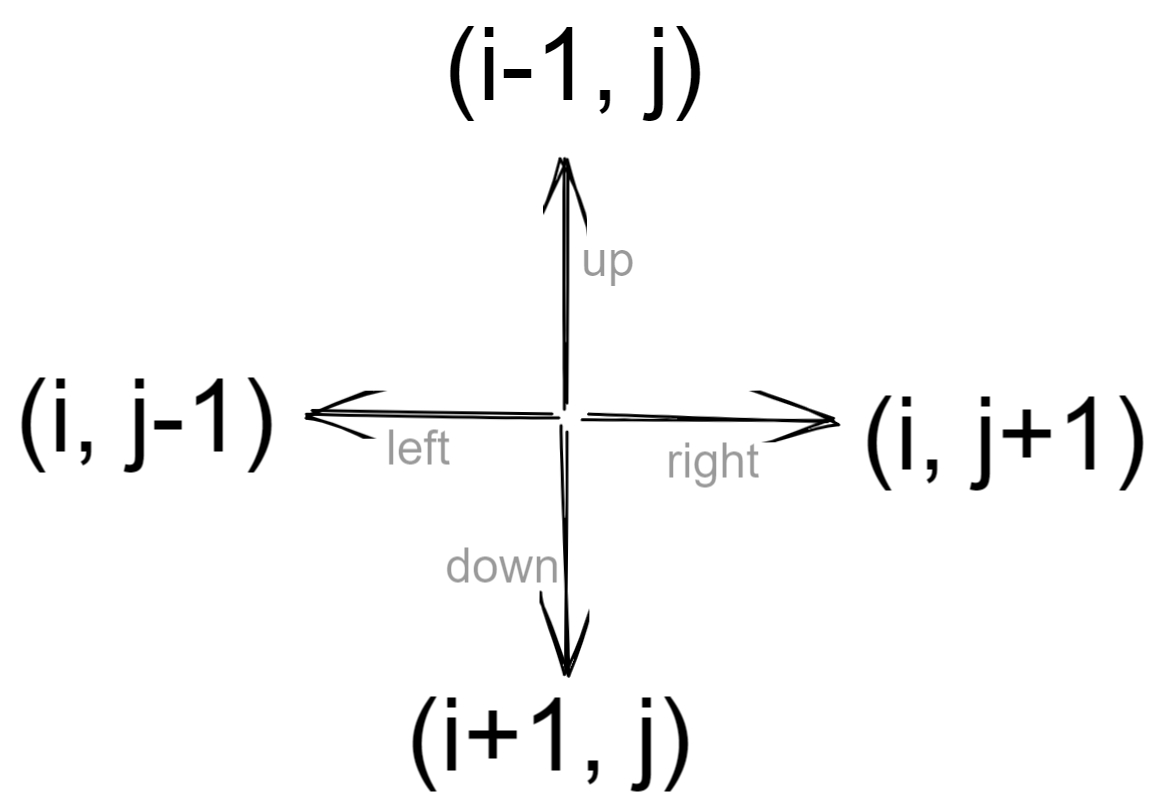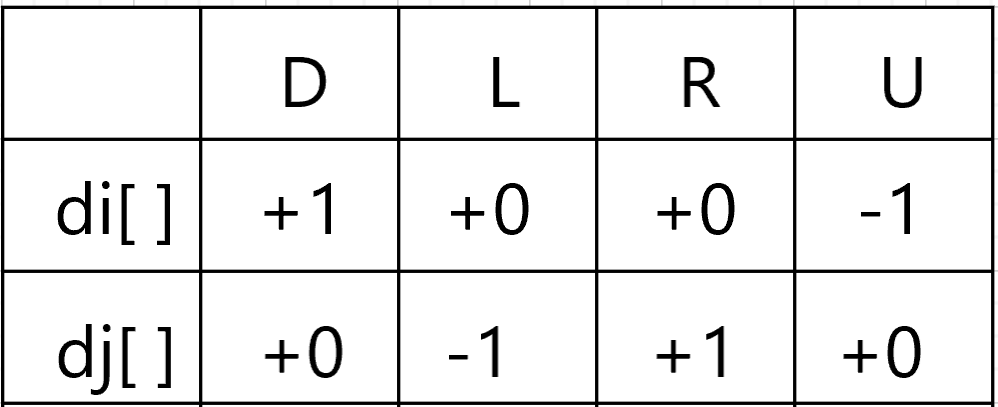## C++ Code

``````#include <bits/stdc++.h>

using namespace std;

class Solution {
void solve(int i, int j, vector < vector < int >> & a, int n, vector < string > & ans, string move,
vector < vector < int >> & vis, int di[], int dj[]) {
if (i == n - 1 && j == n - 1) {
ans.push_back(move);
return;
}
string dir = "DLRU";
for (int ind = 0; ind < 4; ind++) {
int nexti = i + di[ind];
int nextj = j + dj[ind];
if (nexti >= 0 && nextj >= 0 && nexti < n && nextj < n && !vis[nexti][nextj] && a[nexti][nextj] == 1) {
vis[i][j] = 1;
solve(nexti, nextj, a, n, ans, move + dir[ind], vis, di, dj);
vis[i][j] = 0;
}
}

}
public:
vector < string > findPath(vector < vector < int >> & m, int n) {
vector < string > ans;
vector < vector < int >> vis(n, vector < int > (n, 0));
int di[] = {
+1,
0,
0,
-1
};
int dj[] = {
0,
-1,
1,
0
};
if (m == 1) solve(0, 0, m, n, ans, "", vis, di, dj);
return ans;
}
};

int main() {
int n = 4;

vector < vector < int >> m = {{1,0,0,0},{1,1,0,1},{1,1,0,0},{0,1,1,1}};

Solution obj;
vector < string > result = obj.findPath(m, n);
if (result.size() == 0)
cout << -1;
else
for (int i = 0; i < result.size(); i++) cout << result[i] << " ";
cout << endl;

return 0;
}

``````

Output:

DDRDRR DRDDRR

Time Complexity: O(4^(m*n)), because on every cell we need to try 4 different directions.

Space Complexity:  O(m*n) ,Maximum Depth of the recursion tree(auxiliary space).

## Java Code

``````import java.util.*;

// m is the given matrix and n is the order of matrix
class Solution {
private static void solve(int i, int j, int a[][], int n, ArrayList < String > ans, String move,
int vis[][], int di[], int dj[]) {
if (i == n - 1 && j == n - 1) {
return;
}
String dir = "DLRU";
for (int ind = 0; ind < 4; ind++) {
int nexti = i + di[ind];
int nextj = j + dj[ind];
if (nexti >= 0 && nextj >= 0 && nexti < n && nextj < n &&
vis[nexti][nextj] == 0 && a[nexti][nextj] == 1) {

vis[i][j] = 1;
solve(nexti, nextj, a, n, ans, move + dir.charAt(ind), vis, di, dj);
vis[i][j] = 0;

}
}
}
public static ArrayList < String > findPath(int[][] m, int n) {
int vis[][] = new int[n][n];
for (int i = 0; i < n; i++) {
for (int j = 0; j < n; j++) {
vis[i][j] = 0;
}
}
int di[] = {
+1,
0,
0,
-1
};
int dj[] = {
0,
-1,
1,
0
};
ArrayList < String > ans = new ArrayList < > ();
if (m == 1) solve(0, 0, m, n, ans, "", vis, di, dj);
return ans;
}
}
class TUF {
public static void main(String[] args) {

int n = 4;
int[][] a = {{1,0,0,0},{1,1,0,1},{1,1,0,0},{0,1,1,1}};

Solution obj = new Solution();
ArrayList < String > res = obj.findPath(a, n);
if (res.size() > 0) {
for (int i = 0; i < res.size(); i++)
System.out.print(res.get(i) + " ");
System.out.println();
} else {
System.out.println(-1);
}
}
}
``````

Output:

DDRDRR DRDDRR

Time Complexity: O(4^(m*n)), because on every cell we need to try 4 different directions.

Space Complexity:  O(m*n) ,Maximum Depth of the recursion tree(auxiliary space).

Special thanks to Rishiraj Girmal for contributing to this article on takeUforward. If you also wish to share your knowledge with the takeUforward fam, please check out this article